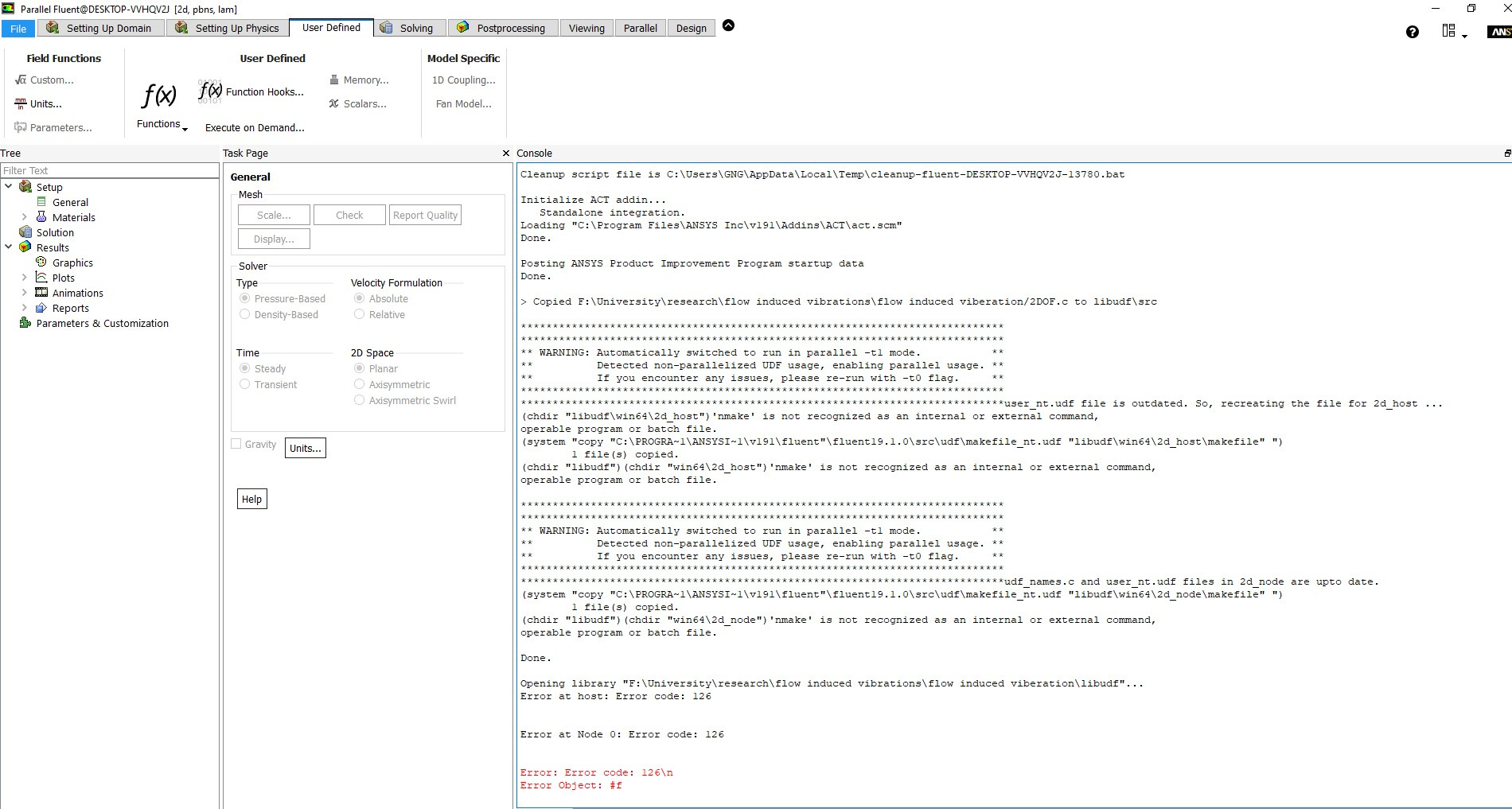## Fluids

Topics relate to Fluent, CFX, Turbogrid and more

•tayyubqayyum0
Subscriberin version 19.1, if you select serial, it means a host node plus a single working node...which essentially makes it parallel computing...to run a true serial case in 19.1, you need to select parallel processing with 'zero' number nodes... so how can I select parallel processing with zero ni get this error when I load UFD n
•Karthik R
Hello,nWhen you launch Fluent, try running it with 0 processors to launch Fluent with a -t0 flag. Please note that this does not work for the recent versions of Fluent there is no serial -t0 launch available. If you are running into this issue, I'd recommend that you work on parallelizing your UDF.nThanks.nKarthikn
•tayyubqayyum0
Subscriber
#include C:\Program Files\ANSYS Inc\v191\fluent\fluent19.1.0\src\udf\udf.hn#include C:\Program Files\ANSYS Inc\v191\fluent\fluent19.1.0\src\mesh\dynamesh_tools.hn#include C:\Program Files\ANSYS Inc\v191\fluent\fluent19.1.0\src\pbns\unsteady.hnnstatic real v_prev; /*velocity in previous time step*/nnDEFINE_SDOF_PROPERTIES(stage, prop, dt, time, dtime)n{n  Thread *t;n  Domain *d = Get_Domain(12);n  n  real cg_y; /*centre of gravity position y*/n  real cg_x; /*centre of gravity position x*/n  real vel_y; /*cylinder velocity y*/n  real vel_x; /*cylinder velocity x*/n  real Fy; /*Lift force*/n  real Fx; /*Drag force*/nn  real mr = 10; /*cylinder mass ratio*/n  real rho = 998.2; /*Fluid Density*/n  real fn = 4.1302676; /*system frequency*/n  real dia = 0.002; /*cylinder diameter*/n  real z = 0; /*Damping coefficient*/n  real cm = 1; /*added mass coefficient*/nn  real mass = (mr*rho*dia*dia*M_PI)/4; /*Cylinder mass*/n  real wn = 2*M_PI*fn; /*system angular velocity*/n  real ky = mass*wn*wn; /*System stiffness y*/n  real kx = mass*wn*wn; /*System stiffness x*/n  real cy = 2*mass*wn*z; /*System damping y*/n  real cx = 2*mass*wn*z; /*System damping*/nn  /*get the thread pointer for which this motion is defined*/n  t = DT_THREAD(dt);nn     prop[SDOF_MASS] = mass; /*system mass of the 6 dof*/n     prop[SDOF_IXX] = (mass*dia*dia)/16;n     prop[SDOF_IYY] = (mass*dia*dia)/16;n     prop[SDOF_IZZ] = (mass*dia*dia)/8;nn     /*limit 4 dofs from 6 dof macro*/n     prop[SDOF_ZERO_TRANS_X] = FALSE;n     prop[SDOF_ZERO_TRANS_Y] = FALSE;n     prop[SDOF_ZERO_TRANS_Z] = TRUE;n     prop[SDOF_ZERO_ROT_X] = TRUE;n     prop[SDOF_ZERO_ROT_Y] = TRUE;n     prop[SDOF_ZERO_ROT_Z] = TRUE;nn      cg_y = DT_CG(dt);n  cg_x = DT_CG(dt);n      vel_y = DT_VEL_CG(dt);n  vel_x = DT_VEL_CG(dt);n      Fy = -ky*cg_y - vel_y*cy;n  Fx = -kx*cg_x - vel_x*cx;nn  prop[SDOF_LOAD_F_Y] = Fy;n  prop[SDOF_LOAD_F_X] = Fx;n} ncan anyone change this for parallel processes ?n
•Karthik R
Hello,nSorry, we will not be able to help you with writing/scripting the UDF in parallel. Please go through the following documentation to parallelize this UDF. Here is the link from our Fluent Customization manual.nHaving said that, if you have any questions related to a macro, we can certainly help here.nThank you.nKarthikn
•YasserSelima
Subscriber
nIt doesn't require any modification to run in parallel. But are you sure of Get_Domain(12) ??n
•YasserSelima
Subscriber
Your problem is not in parallelizing the UDF. it is something in the setting. nmake is not recognized .. the file is not compiled, neither for serial or parallel ... n
•tayyubqayyum0
Subscriber
Thanks for help it's okay now n
•YasserSelima
Subscriber
you are welcome!n